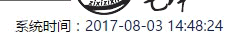## JavaScript 模糊查询字符串/数组、日期时间格式化等方法 有更新！

2017-05-31
4 评论 8,188 浏览

### 1. JavaScript 正则表达式筛选字符串/元素内容

#### js 源码

``````/**
* 为字符串添加模糊比较的方法
* @param exp 模糊查询字符串，支持正则表达式
* @param i 是否区分大小写
* @returns
*/
String.prototype.isLike = function(exp, i) {
var str = this;
i = i == null ? false : i;
if (exp.constructor == String) {
/* 首先将表达式中的‘_’替换成‘.’，但是‘[_]’表示对‘_’的转义，所以做特殊处理 */
var s = exp.replace(/_/g, function(m, i) {
if (i == 0 || i == exp.length - 1) {
return ".";
} else {
if (exp.charAt(i - 1) == "[" && exp.charAt(i + 1) == "]") {
return m;
}
return ".";
}
});
/* 将表达式中的‘%’替换成‘.’，但是‘[%]’表示对‘%’的转义，所以做特殊处理 */
s = s.replace(/%/g, function(m, i) {
if (i == 0 || i == s.length - 1) {
return ".*";
} else {
if (s.charAt(i - 1) == "[" && s.charAt(i + 1) == "]") {
return m;
}
return ".*";
}
});

/*将表达式中的‘[_]’、‘[%]’分别替换为‘_’、‘%’*/
s = s.replace(/\[_\]/g, "_").replace(/\[%\]/g, "%");

/*对表达式处理完后构造一个新的正则表达式，用以判断当前字符串是否和给定的表达式相似*/
var regex = new RegExp("" + s, i ? "" : "i");
return regex.test(this);
}
return false;
};
``````

#### 使用方法：### 2. JavaScript 模糊检索数组内容

#### js 源码

``````/**
* 为数组添加模糊查询方法
* @param exp 正则表达式字符串
* @param fun 自定义过滤方法
* @returns 返回 exp 匹配到的位置
*/
Array.prototype.selectLike = function(exp, fun) {
var arr = [];
if (fun && fun.constructor == Function) { // 使用自定义的过滤方法
for (var i = 0; i < this.length; i++) {
if (fun(this[i], exp)) {
arr.push(i);
}
}
} else {
for (var i = 0; i < this.length; i++) {
if (this[i].isLike(exp, false)) { // 使用上面 String 对象的 isLike 方法
arr.push(i); // 匹配到的数组下标位置
// arr.push(this[i]); // 所有匹配到的数组内容
// return this[i]; // 匹配到第一个就返回当前数组内容
}
}
}
return arr;
};
``````

#### 使用方法：### 3. JavaScript 日期格式化

#### js 源码

``````/**
* 日期格式化
* @param {Object} format 格式化字符串，如：yyyy-MM-dd hh:mm:ss.S
* @memberOf {TypeName}
* @return {TypeName} 格式化后的时间：2016-03-08 13:29:27.344
* @author Tanken·L
*/
Date.prototype.format = function(format) {
format = format == null ? "yyyy-MM-dd hh:mm:ss.S" : format;
var o = {
"M+": this.getMonth() + 1, //月
"d+": this.getDate(), //天
"h+": this.getHours(), //小时
"m+": this.getMinutes(), //分钟
"s+": this.getSeconds(), //秒钟
"q+": Math.floor((this.getMonth() + 3) / 3), //季度
"S": this.getMilliseconds() //毫秒
}
// y|Y：格式化年份
if (/((y|Y)+)/.test(format)) {
format = format.replace(RegExp.\$1, (this.getFullYear() + "").substr(4 - RegExp.\$1.length));
}
// w|W：格式化星期
if (/((w|W)+)/.test(format)) {
var weekMin = ['日', '一', '二', '三', '四', '五', '六'];
var weekMid = ['周日', '周一', '周二', '周三', '周四', '周五', '周六'];
var weekMax = ['星期日', '星期一', '星期二', '星期三', '星期四', '星期五', '星期六'];
var strLen = RegExp.\$1.length;
var weeks = strLen == 1 ? weekMin : strLen == 2 ? weekMid : weekMax;
format = format.replace(RegExp.\$1, weeks[this.getDay()]);
}
for (var k in o) {
if (new RegExp("(" + k + ")").test(format)) {
format = format.replace(RegExp.\$1, RegExp.\$1.length == 1 ? o[k] : ("00" + o[k]).substr(("" + o[k]).length));
}
}
return format;
}
``````

#### 使用方法：

``````new Date().format("yyyy-MM-dd hh:mm:ss.S WW"); // 返回 "2017-05-31 21:32:30.289 周三"
``````

### 4. JavaScript 判断闰年

#### js 源码

``````/**
* 判断闰年
*/
Date.prototype.isLeapYear = function() {
return (0 == this.getYear() % 4 && ((this.getYear() % 100 != 0) || (this.getYear() % 400 == 0)));
}
``````

#### 使用方法：

``````new Date().isLeapYear(); // true | false
new Date("2017").isLeapYear(); // return false
new Date("2020").isLeapYear(); // return true
``````

### 5. JavaScript 日期加减天数运算

#### js 源码

``````/**
* 日期加减天数运算
* @param {Object} idate 传入日期
* @param {Object} addDays 要加的天数，可以为负数
* @return {TypeName} 计算后的时间
* @author Tanken·L
*/
function aroundDate(idate, addDays) {
idate = idate == null ? new Date() : idate;
idate = idate.valueOf(); // 日期转为毫秒数
idate = idate + (addDays * 24 * 60 * 60 * 1000);
idate = new Date(idate);
return idate;
}
``````

#### 使用方法：

``````aroundDate(new Date(), 1).format("yyyy-MM-dd hh:mm:ss.S"); // 返回 "2017-06-01 22:00:53.728"
``````

### 6. JavaScript 获取指定月份的天数

#### js 源码

``````/**
* 返回指定月份的天数
* @param {Object} year  某年
* @param {Object} month 某月
* @return {TypeName} 某月的天数
* @author Tanken·L
*/
function dayCountOfMonth(year, month) {
year = year == null ? new Date().getFullYear() : year;
month = month == null ? new Date().getMonth() + 1 : month;
return new Date(year, month, 0).getDate();
}
``````

#### 使用方法：

``````dayCountOfMonth(); // 返回当前月份天数
dayCountOfMonth(2017, 2); // 返回 28
``````

### 7. JavaScript 在网页显示实时时间

#### js 源码

``````/**
* 在指定元素内动态显示时间
* @selector 元素 id 选择器（若使用 jQuery，可以是其他选择器）
* @useCn 是否格式化为中文年月日
* @timeStr 传入时间戳
* @author Tanken·L
*/
function showNowTime(selector, useCn, datetime) {
var nowTime = datetime == null ? new Date() : new Date(datetime);
var year = nowTime.getFullYear(),
month = nowTime.getMonth() + 1,
date = nowTime.getDate(),
hour = nowTime.getHours(),
minute = nowTime.getMinutes(),
second = nowTime.getSeconds();
if(parseInt(month) < 10) { month = "0" + month; }
if(parseInt(date) < 10) { date = "0" + date; }
if(parseInt(hour) < 10) { hour = "0" + hour; }
if(parseInt(minute) < 10) { minute = "0" + minute; }
if(parseInt(second) < 10) { second = "0" + second; }
var time = year + "/" + month + "/" + date + " " + hour + ":" + minute + ":" + second;
time = Date.parse(time) + 1000;
// 显示时间
document.getElementById(selector).innerHTML(year + (useCn ? "年" : "-") + month + (useCn ? "月" : "-") + date + (useCn ? "日 " : " ") + hour + ":" + minute + ":" + second);
setTimeout("showNowTime('" + selector + "', " + useCn + ", " + time + ")", 998); // 由于计算耗时，使用 1000 每 5 分钟约延迟 2 分钟
}
``````

#### 使用方法：

``````showNowTime("now-time", false, Date.parse("2017/08/03 14:58:14")); // 传入指定时间，如网络服务器时间，格式为 yyyy/MM/dd HH:mm:ss，显示为 yyyy-MM-dd HH:mm:ss
showNowTime("now-time", true); // 当前客户端系统时间，显示为 yyyy年MM月dd日 HH:mm:ss
``````1. 使用移动设备访问，复制后转到电脑；
2. 开发者工具 👉 选中代码区域 👉 `Copy selector` 👉 \$(selector).text(); 👉 回车见证奇迹吧！作者：星柒天 出处：https://zixizixi.cn/ 本文发布于 子兮子兮 博客，欢迎转载，但未经作者同意请保留此声明，并在文章页面明显位置给出原文连接。 本作品采用知识共享署名-相同方式共享 4.0 国际许可协议进行许可。

•• 为什么不是工具公主

• 嘿嘿，都是平时开发用到的• 你收藏了这么多代码啊，工具王子你好